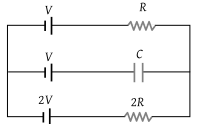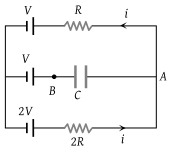# NEET Physics Current Electricity Questions SolvedIn the given circuit, with steady current, the potential drop across the capacitor must be(1) V

(2) V / 2

(3) V / 3

(4) 2V / 3

(3) Moving anticlockwise from A$-\text{\hspace{0.17em}}iR-V+2V-2iR=0$

or $3iR=V$ or $i=\frac{V}{3R}$

${V}_{A}-{V}_{B}=iR+V-V=iR$

⇒ Potential drop across C = $\frac{V}{3}$

Difficulty Level:

• 17%
• 36%
• 36%
• 14%
Crack NEET with Online Course - Free Trial (Offer Valid Till September 24, 2019)